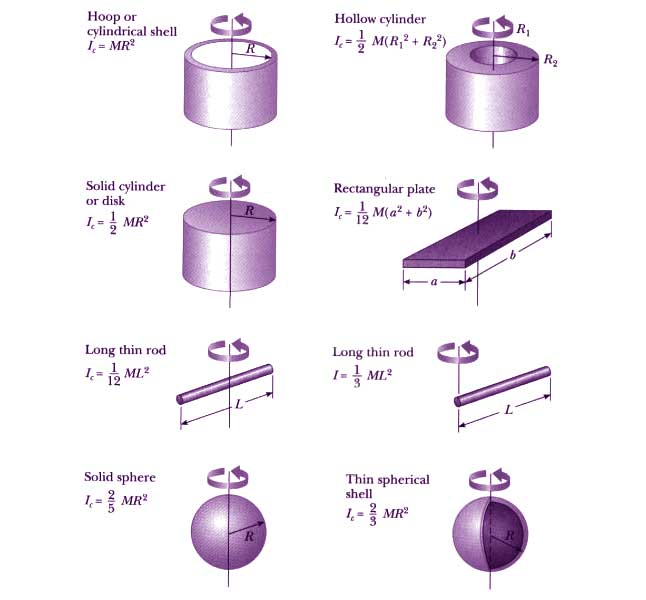# Moment Of Inertia Formula

Moment of Inertia is a measure of the amount of moment provided to a body to conquer its own inertia. In other words it is defined as the quantity which describes the body’s tendency to resist angular acceleration. It describes about the object offering resistance to accelerate or slow down its own motion.

It is the sum of the products of the mass of every component in the body with the square of the distance from the point of rotation.

## Moment Of Inertia Formula

 General form $I=mr^{2}$ Integral form $I=\int dI=\int_{0}^{M}r^{2}dm$

### Notations Used In Moment Of Inertia Formula

• r is the distance between the axis and rotation in m
• m is the mass of the object in kg
• I is the moment of Inertia in kg.m²

### Moment Of Inertia Units And Dimension

 SI unit kg.m2 Imperial system unit lbf.ft.s2 Dimensional formula ML2

### Moment Of Inertia For Other Rigid Bodies And Solved ExamplesExample 1

Determine the Moment of inertia of the ball having mass of 10 Kg and radius of 5 cm.

Solution:

Given parameters are,

Mass of the ball = 10 Kg,

Radius = 5 cm

= 0.05 m,

Moment of Inertia is given as

I = MR²

= 10 × (0.05 )²

= 0.025 Kgm²

Example 2

A sphere is moving around and its moment of inertia is 20 Kgm² and radius 1 m, determine its mass.

Solution:

Given:

Moment of inertia, I = 20 Kgm²,

Radius of sphere, R = 1 m,

Moment of Inertia formula is given by,

I = MR²

M = I / R²

= 20 / 1

= 20 Kg

To learn more on the moment of inertia derivation, stay tuned with BYJU’S.

#### Practise This Question

An oil drop has 7.99 × 1019 C charge. How many electrons does this oil drop has?